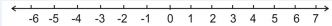1. /
2. CBSE
3. /
4. Class 09
5. /
6. Number Systems class 9...

# Number Systems class 9 Notes Mathematics

CBSE class 9 Mathematics Chapter 1 Number Systems notes in PDF are available for free download in myCBSEguide mobile app. The best app for CBSE students now provides Number Systems class 9 Notes latest chapter wise notes for quick preparation of CBSE exams and school based annual examinations. Class 9 Mathematics notes on Chapter 1 Number Systems are also available for download in CBSE Guide website.

## CBSE Guide Number Systems class 9 Notes

CBSE guide notes are the comprehensive notes which covers the latest syllabus of CBSE and NCERT. It includes all the topics given in NCERT class 9 Mathematics text book. Users can download CBSE guide quick revision notes from myCBSEguide mobile app and my CBSE guide website.

## 9 Mathematics notes Chapter 1 Number Systems

Download CBSE class 9th revision notes for Chapter 1 Number Systems in PDF format for free. Download revision notes for Number Systems class 9 Notes and score high in exams. These are the Number Systems class 9 Notes prepared by team of expert teachers. The revision notes help you revise the whole chapter in minutes. Revising notes in exam days is on of the best tips recommended by teachers during exam days.

CBSE Class 09 Mathematics
Revision Notes
CHAPTER – 1
NUMBER SYSTEMS

1 Rational Numbers

2 Irrational Numbers

3 Real Numbers and their Decimal Expansions

4 Operations on Real Numbers

5 Laws of Exponents for Real Numbers• Natural numbers are : 1, 2, 3, …………….. denoted by N.
• Whole numbers are : 0, 1, 2, 3, ……………… denoted by W.
• Integers : ……. -3, -2, -1, 0, 1, 2, 3, ……………… denoted by Z.
• Rational numbers – All the numbers which can be written in the form  are called rational numbers where p and q are integers and  Every integer p is also a rational number, can be written as
• Irrational numbers – A number is called irrational, if it cannot be written in the form   where p and q are integers and
• The decimal expansion of a rational number is either terminating or non terminating recurring. Thus we say that a number whose decimal expansion is either terminating or non terminating recurring is a rational number.
• Terminating decimals: The rational numbers with a finite decimal part or for which the long division terminates after a finite number of steps are known as finite or terminating decimals.
• Non-Terminating decimals: The rational numbers with an infinite decimal part or for which the long division does not terminate even after an infinite number of steps are known as infinite or non-terminating decimals
• The decimal expansion of a irrational number is non terminating non recurring.
• All the rational numbers and irrational numbers taken together make a collection of real numbers.
• A real number is either rational or irrational.
• If r is rational and s is irrational then r+s, r–s, r.s are always irrational numbers but  may be rational or irrational.
• If n is a natural number other than a perfect square, then  is a irrational number.
• Negative of an irrational number is an irrational number.
• There is a real number corresponding to every point on the number line. Also, corresponding to every real number there is a point on the number line.
• Every irrational number can be represented on a number line using Pythagoras theorem.
• For every positive real number   can be represented by a point on the number line by using the following steps:
1. Obtain all positive real numbers  (say).
2. Draw a line and mark a point P on it.
3. Make a point Q on the line such that PQ =  units.
4. From point Q marka distance of 1 unit and mark the new point as R.
5. Find the mid-point of PR and mark the point as O.
6. Draw a circle with centre O and radius OR.
7. Draw a line perpendicular to PR passing through Q and intersecting the semi-circle at S. Length QS is equal to
• Rationalization means to remove square root from the denominator.

to remove we will multiply both numerator & denominator by

its rationalization factor

## Number Systems class 9 Notes

• CBSE Revision notes for Class 9 Mathematics PDF
• CBSE Revision notes Class 9 Mathematics – CBSE
• CBSE Revisions notes and Key Points Class 9 Mathematics
• Summary of the NCERT books all chapters in Mathematics class 9
• Short notes for CBSE class 9th Mathematics
• Key notes and chapter summary of Mathematics class 9
• Quick revision notes for CBSE board exams

## CBSE Class-9 Revision Notes and Key Points

Number Systems class 9 Notes. CBSE quick revision note for Class-9 Mathematics, Chemistry, Maths, Biology and other subject are very helpful to revise the whole syllabus during exam days. The revision notes covers all important formulas and concepts given in the chapter. Even if you wish to have an overview of a chapter, quick revision notes are here to do if for you. These notes will certainly save your time during stressful exam days.

To download Number Systems class 9 Notes, sample paper for class 9 Mathematics, Social Science, Science, English Communicative; do check myCBSEguide app or website. myCBSEguide provides sample papers with solution, test papers for chapter-wise practice, NCERT solutions, NCERT Exemplar solutions, quick revision notes for ready reference, CBSE guess papers and CBSE important question papers. Sample Paper all are made available through the best app for CBSE students and myCBSEguide website.Test Generator

Create question paper PDF and online tests with your own name & logo in minutes.myCBSEguide

Question Bank, Mock Tests, Exam Papers, NCERT Solutions, Sample Papers, Notes